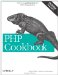# Recipe 2.13. Doing Trigonometry in Degrees, Not Radians

#### 2.13.1. Problem

You have numbers in degrees but want to use the trigonometric functions.

#### 2.13.2. Solution

`\$cosine = cos(deg2rad(\$degree));`

#### 2.13.3. Discussion

By definition, 360 degrees is equal to 2Π radians, so it's easy to manually convert between the two formats. However, these functions use PHP's internal value of Π, so you're assured a high-precision answer. To access this number for other calculations, use the constant M_PI, which is 3.14159265358979323846.

There is no built-in support for radians. This is considered a feature, not a bug.PHP Cookbook: Solutions and Examples for PHP Programmers
ISBN: 0596101015
EAN: 2147483647
Year: 2006
Pages: 445

Similar book on Amazon